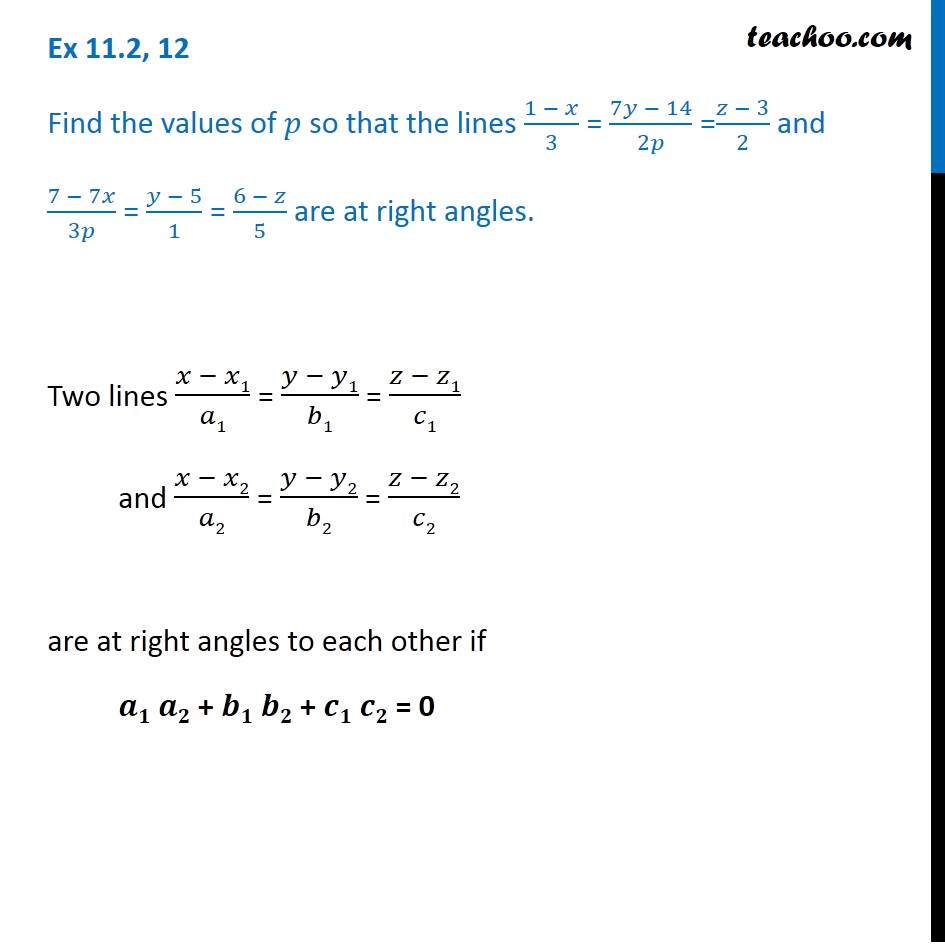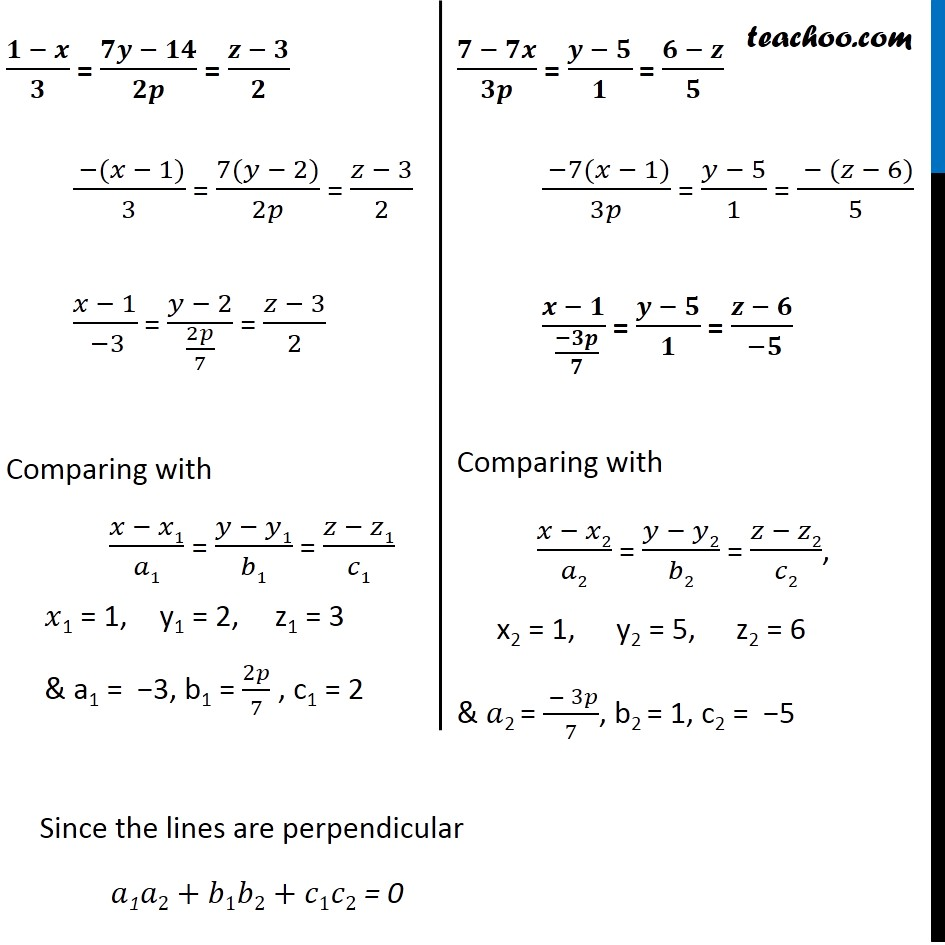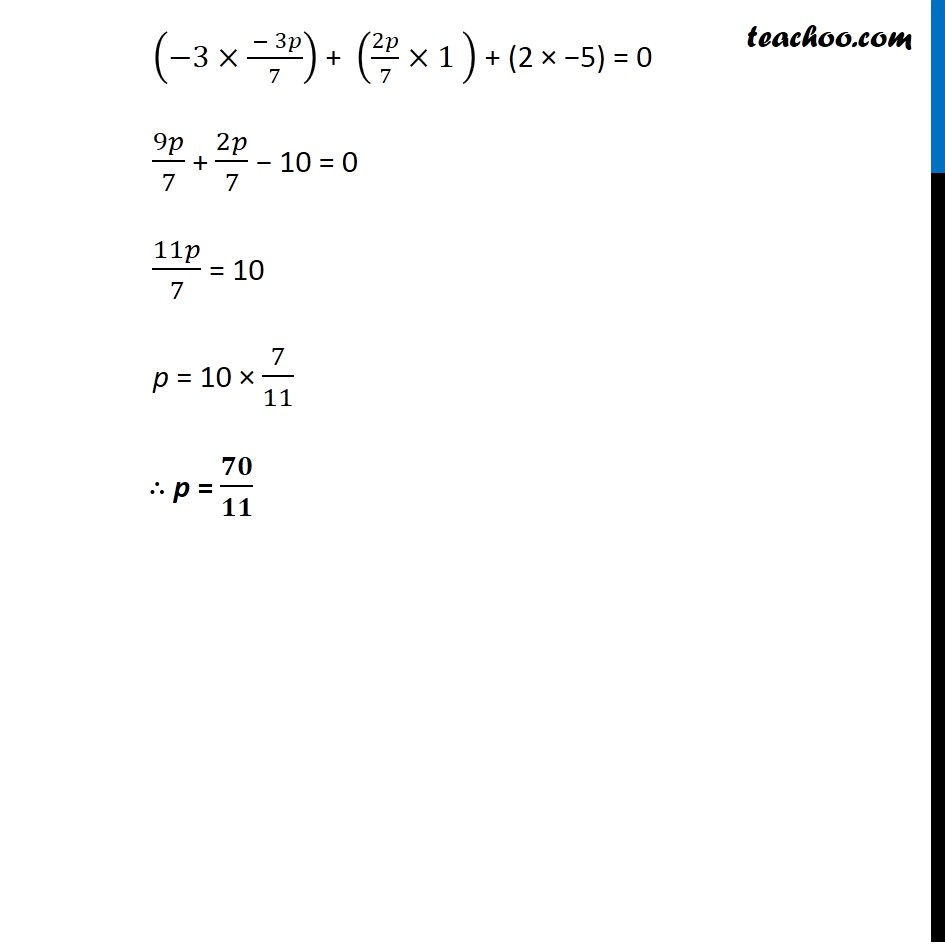Ex 11.2

Chapter 11 Class 12 Three Dimensional Geometry (Term 2)
Serial order wise### Transcript

Ex 11.2, 12 Find the values of 𝑝 so that the lines (1 − 𝑥)/3 = (7𝑦 − 14)/2𝑝 =(𝑧 − 3)/2 and (7 − 7𝑥)/3𝑝 = (𝑦 − 5)/1 = (6 − 𝑧)/5 are at right angles. Two lines (𝑥 − 𝑥1)/𝑎1 = (𝑦 − 𝑦1)/𝑏1 = (𝑧 − 𝑧1)/𝑐1 and (𝑥 − 𝑥2)/𝑎2 = (𝑦 − 𝑦2)/𝑏2 = (𝑧 − 𝑧2)/𝑐2 are at right angles to each other if 𝒂𝟏 𝒂𝟐 + 𝒃𝟏 𝒃𝟐 + 𝒄𝟏 𝒄𝟐 = 0 (𝟏 − 𝒙)/𝟑 = (𝟕𝒚 − 𝟏𝟒)/𝟐𝒑 = (𝒛 − 𝟑)/𝟐 ( −(𝑥 − 1))/3 = (7(𝑦 − 2))/2𝑝 = (𝑧 − 3)/2 (𝑥 − 1)/( −3) = (𝑦 − 2)/(2𝑝/7) = (𝑧 − 3)/2 Comparing with (𝑥 − 𝑥1)/𝑎1 = (𝑦 − 𝑦1)/𝑏1 = (𝑧 − 𝑧1)/𝑐1 𝑥1 = 1, y1 = 2, z1 = 3 & a1 = −3, b1 = 2𝑝/7 , c1 = 2 (𝟕 − 𝟕𝒙)/𝟑𝒑 = (𝒚 − 𝟓)/𝟏 = (𝟔 − 𝒛)/𝟓 ( −7(𝑥 − 1))/3𝑝 = (𝑦 − 5)/1 = ( − (𝑧 − 6))/5 (𝒙 − 𝟏)/( (−𝟑𝒑)/𝟕) = (𝒚 − 𝟓)/𝟏 = (𝒛 − 𝟔)/( −𝟓) Comparing with (𝑥 − 𝑥2)/𝑎2 = (𝑦 − 𝑦2)/𝑏2 = (𝑧 − 𝑧2)/𝑐2, x2 = 1, y2 = 5, z2 = 6 & 𝑎2 = ( − 3𝑝)/7, b2 = 1, c2 = −5 Since the lines are perpendicular 𝑎1𝑎2+𝑏1𝑏2+𝑐1𝑐2 = 0 (−3×( − 3𝑝)/7) + (2𝑝/7×1 ) + (2 × −5) = 0 9𝑝/7 + 2𝑝/7 − 10 = 0 11𝑝/7 = 10 p = 10 × 7/11 ∴ p = 𝟕𝟎/𝟏𝟏